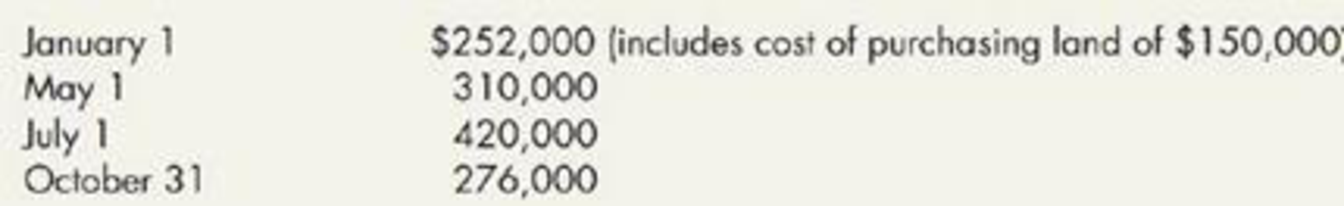Chapter 10, Problem 16E### Intermediate Accounting: Reporting...

3rd Edition
James M. Wahlen + 2 others
ISBN: 9781337788281

#### Solutions

Chapter
Section### Intermediate Accounting: Reporting...

3rd Edition
James M. Wahlen + 2 others
ISBN: 9781337788281
Textbook Problem
153 views

# Interest During Construction Matrix Inc. borrowed $1,000,000 at 8% to finance the construction of a new building for its own use. Construction began on January 1, 2019, and was completed on October 31, 2019. Expenditures related to this building were:In addition, Matrix had additional debt (unrelated to the construction) of$500,000 at 9% and $800,000 at 10%. All debt was outstanding for the entire year.Required: 1. Compute the amount of interest capitalized related to the construction of the building. 2. If the expenditures are assumed to have been incurred evenly throughout the year, compute weighted average accumulated expenditures and the amount of interest capitalized on the building. 1. To determine Calculate the amount of interest capitalized related to the construction of the building. Explanation Weighted average interest rate: Weighted average interest rate is the total of the construction expenses weighted by the measure of time that interest cost is acquired on those expenditures during the period of construction. Capitalized interest: Interest Cost incurred to finance the construction of a long-term construction projects are known as capitalized interest. Capitalized interest-construction loan of$1,000,000:

Step 1:

 Expenditures × Portion of  year outstanding = Weighted average accumulated expenditures January 1 $252,000 × 10/12 (January 1-October 31) =$210,000 May 1 $310,000 × 6/12 (May 1-October 31) =$155,000 July 1 $420,000 × 4/12 (July1-October 31) =$140,000 October 31 $276,000 × 0/12 (October 31-October 31) =$0 $1,258,000$505,000

Table (1)

Note: Interests costs (if any) capitalized are included as a part of the cost of the building, not the part of the land.

Step 2:

Interest rate is 8%...

2.

To determine

Calculate the weighted average accumulated expenditure and the amount of interest capitalized on the building.

### Still sussing out bartleby?

Check out a sample textbook solution.

See a sample solution

#### The Solution to Your Study Problems

Bartleby provides explanations to thousands of textbook problems written by our experts, many with advanced degrees!

Get Started

#### A stock had a 12% return last year, a year when the overall stock market decline. Does this mean that the stock...

Fundamentals of Financial Management, Concise Edition (with Thomson ONE - Business School Edition, 1 term (6 months) Printed Access Card) (MindTap Course List)

#### Owners of corporations have no personal liability for debts of the corporation.

College Accounting, Chapters 1-27 (New in Accounting from Heintz and Parry)